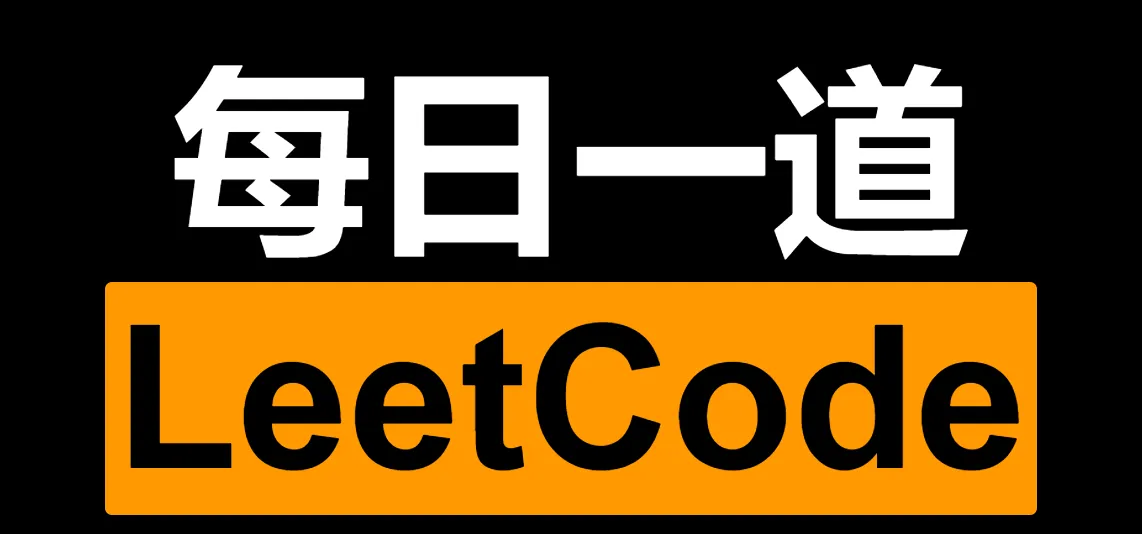# leetcode 153. Find Minimum in Rotated Sorted Array 寻找旋转排序数组中的最小值 (中)

• 2022 年 5 月 28 日
• 本文字数：925 字

阅读完需：约 3 分钟# 一、题目大意

• n == nums.length

• 1 <= n <= 5000

• -5000 <= nums[i] <= 5000

• nums 中的所有整数 互不相同

• nums 原来是一个升序排序的数组，并进行了 1 至 n 次旋转

# 三、解题方法

## 3.1 Java 实现

public class Solution {    public int findMin(int[] nums) {        int l = 0;        int r = nums.length - 1;        int min = nums;        while (l <= r) {            int mid = l + (r - l) / 2;            // 判断有序区间            if (nums[mid] >= nums[l]) {                // 左侧有序                min = Math.min(nums[l], min);                l = mid + 1;            } else {                // 右侧有序                min = Math.min(nums[mid], min);                r = mid - 1;            }        }        return min;    }}

# 四、总结小记

• 2022/5/28 二分查找，循环结束条件和内部比较时到底加不加等号老是分不清，每次都得通过调试## 评论